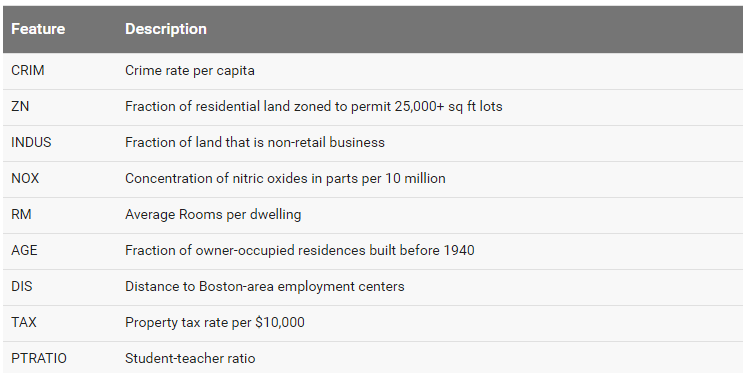# learn创建输入函数的不二等秘书籍

1. 如何使用input_fn自定义输入管道

``````# 将特征与标签数据载入
filename=IRIS_TRAINING, target_dtype=np.int, features_dtype=np.float32)

filename=IRIS_TEST, target_dtype=np.int, features_dtype=np.float32)

# 然后将两个数据喂给.fit()函数去训练
classifier.fit(x=training_set.data,
y=training_set.target,
steps=2000)
``````

1.1 解剖input_fn函数的结构

``````def my_input_fn():

# ...then return 1) a mapping of feature columns to Tensors with
# the corresponding feature data, and 2) a Tensor containing labels
# 然后返回新的特征数据与标签数据（都是以tensor的形式）
return feature_cols, labels
``````

（1）处理后的特征：feature_cols，格式是一个map，key是特征的名称，value是tensor形式的对应的特征列数据
（2）标签数据：labels，一个包含标签数据的tensor

1.2 如何将特征数据转换成tensors形式

``````feature_column_data = [1, 2.4, 0, 9.9, 3, 120]
feature_tensor = tf.constant(feature_column_data)
``````

``````sparse_tensor = tf.SparseTensor(indices=[[0,1], [2,4]],
values=[6, 0.5],
dense_shape=[3, 5])
``````

（1）dense_shape

（2）indices

(3)values

value是一个1维的tensor,

[2,4]]，values=[18,
3.6]，表示在行索引为1列索引为3的位置值为18，在行索引为2列索引为4的位置值为3.6

[[0, 6, 0, 0, 0]
[0, 0, 0, 0, 0]
[0, 0, 0, 0, 0.5]]

1.3 如何将input_fn数据传给模型

``````classifier.fit(input_fn=my_input_fn, steps=2000)
``````

``````classifier.fit(input_fn=my_input_fn(training_set), steps=2000)
``````

（1）再写一个封装函数如下：

``````def my_input_function_training_set():
return my_input_function(training_set)

classifier.fit(input_fn=my_input_fn_training_set, steps=2000)
``````

（2）使用Python’s functools.partial方法：

``````classifier.fit(input_fn=functools.partial(my_input_function,
data_set=training_set), steps=2000)
``````

（3）在lambda中调用输入函数，然后将参数传入input_fn中

``````classifier.fit(input_fn=lambda: my_input_fn(training_set), steps=2000)
``````

2.案例实战

2.1 数据介绍boston_test.csv（测试集）, and boston_predict.csv（预测集）这份文件

2.2 加载数据

verbosity为INFO，这样就可以获取到更多的日志信息了。

``````from __future__ import absolute_import
from __future__ import division
from __future__ import print_function

import itertools

import pandas as pd
import tensorflow as tf

tf.logging.set_verbosity(tf.logging.INFO)
``````

``````COLUMNS = ["crim", "zn", "indus", "nox", "rm", "age",
"dis", "tax", "ptratio", "medv"]
FEATURES = ["crim", "zn", "indus", "nox", "rm",
"age", "dis", "tax", "ptratio"]
LABEL = "medv"
``````

``````training_set = pd.read_csv("boston_train.csv", skipinitialspace=True,
skiprows=1, names=COLUMNS)

skiprows=1, names=COLUMNS)

skiprows=1, names=COLUMNS)
``````

2.3 定义特征列并且创建回归模型

``````feature_cols = [tf.contrib.layers.real_valued_column(k)
for k in FEATURES]
``````

1. feature_columns：一组刚刚定义的特征列
2. hidden_units：每层隐藏层的神经网络个数
3. model_dir：模型保存的路径
``````regressor = tf.contrib.learn.DNNRegressor(feature_columns=feature_cols,
hidden_units=[10, 10],
model_dir="/tmp/boston_model")
``````

2.4 构建输入函数input_fn

``````def input_fn(data_set):
feature_cols = {k: tf.constant(data_set[k].values)
for k in FEATURES}
labels = tf.constant(data_set[LABEL].values)
return feature_cols, labels
``````

2.5 训练模型

``````regressor.fit(input_fn=lambda: input_fn(training_set), steps=5000)
``````

INFO:tensorflow:Step 1: loss = 483.179
INFO:tensorflow:Step 101: loss = 81.2072
INFO:tensorflow:Step 201: loss = 72.4354

INFO:tensorflow:Step 1801: loss = 33.4454
INFO:tensorflow:Step 1901: loss = 32.3397
INFO:tensorflow:Step 2001: loss = 32.0053
INFO:tensorflow:Step 4801: loss = 27.2791
INFO:tensorflow:Step 4901: loss = 27.2251
INFO:tensorflow:Saving checkpoints for 5000 into
/tmp/boston_model/model.ckpt.
INFO:tensorflow:Loss for final step: 27.1674.

2.6 评估模型

``````ev = regressor.evaluate(input_fn=lambda: input_fn(test_set), steps=1)
``````

``````loss_score = ev["loss"]
print("Loss: {0:f}".format(loss_score))
``````

INFO:tensorflow:Eval steps [0,1) for training step 5000.
INFO:tensorflow:Saving evaluation summary for 5000 step: loss =
11.9221
Loss: 11.922098

2.7 使用模型做预测

``````y = regressor.predict(input_fn=lambda: input_fn(prediction_set))

# .predict() returns an iterator; convert to a list and print predictions

predictions = list(itertools.islice(y, 6))
print ("Predictions: {}".format(str(predictions)))
``````

Predictions: [ 33.30348587  17.04452896  22.56370163  34.74345398
14.55953979
19.58005714]